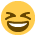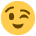# Two problems

Posts: 1,876,324 The Mix Honorary Guru
1) The fonts and sizes boxes are broken, you just get the coding text not the options.

2) The option to hide certain parts of the board has gone with the new layouts. Not a massive one but I really dont use a couple of parts of the forum.

• TheSite Graduate Posts: 18,265 Incredible Poster
Arial
Arial Black
Arial Narrow
Book Antiqua
Century Gothic
Comic Sans MS
Courier New
Fixedsys
Franklin Gothic Medium
Garamond
Georgia
Impact
Lucida Console
Lucida Sans Unicode
Microsoft Sans Serif
Palatino Linotype
System
Tahoma
Times New Roman
Trebuchet MS
Verdana
function (iterator, context) { var index = 0; iterator = iterator.bind(context); try { this._each(function (value) {iterator(value, index++);}); } catch (e) { if (e != \$break) { throw e; } } return this; }
function (number, iterator, context) { iterator = iterator ? iterator.bind(context) : Prototype.K; var index = - number, slices = [], array = this.toArray(); while ((index += number) < array.length) { slices.push(array.slice(index, index + number)); } return slices.collect(iterator, context); }
function (iterator, context) { iterator = iterator ? iterator.bind(context) : Prototype.K; var result = true; this.each(function (value, index) {result = result && !!iterator(value, index);if (!result) {throw \$break;}}); return result; }
function (iterator, context) { iterator = iterator ? iterator.bind(context) : Prototype.K; var result = false; this.each(function (value, index) {if (result = !!iterator(value, index)) {throw \$break;}}); return result; }
function (iterator, context) { iterator = iterator ? iterator.bind(context) : Prototype.K; var results = []; this.each(function (value, index) {results.push(iterator(value, index));}); return results; }
function (iterator, context) { iterator = iterator.bind(context); var result; this.each(function (value, index) {if (iterator(value, index)) {result = value;throw \$break;}}); return result; }
function (iterator, context) { iterator = iterator.bind(context); var results = []; this.each(function (value, index) {if (iterator(value, index)) {results.push(value);}}); return results; }
function (filter, iterator, context) { iterator = iterator ? iterator.bind(context) : Prototype.K; var results = []; if (Object.isString(filter)) { filter = new RegExp(filter); } this.each(function (value, index) {if (filter.match(value)) {results.push(iterator(value, index));}}); return results; }
function (object) { if (Object.isFunction(this.indexOf)) { if (this.indexOf(object) != -1) { return true; } } var found = false; this.each(function (value) {if (value == object) {found = true;throw \$break;}}); return found; }
function (number, fillWith) { fillWith = Object.isUndefined(fillWith) ? null : fillWith; return this.eachSlice(number, function (slice) {while (slice.length < number) {slice.push(fillWith);}return slice;}); }
function (memo, iterator, context) { iterator = iterator.bind(context); this.each(function (value, index) {memo = iterator(memo, value, index);}); return memo; }
function (method) { var args = \$A(arguments).slice(1); return this.map(function (value) {return value[method].apply(value, args);}); }
function (iterator, context) { iterator = iterator ? iterator.bind(context) : Prototype.K; var result; this.each(function (value, index) {value = iterator(value, index);if (result == null || value >= result) {result = value;}}); return result; }
function (iterator, context) { iterator = iterator ? iterator.bind(context) : Prototype.K; var result; this.each(function (value, index) {value = iterator(value, index);if (result == null || value < result) {result = value;}}); return result; }
function (iterator, context) { iterator = iterator ? iterator.bind(context) : Prototype.K; var trues = [], falses = []; this.each(function (value, index) {(iterator(value, index) ? trues : falses).push(value);}); return [trues, falses]; }
function (property) { var results = []; this.each(function (value) {results.push(value[property]);}); return results; }
function (iterator, context) { iterator = iterator.bind(context); var results = []; this.each(function (value, index) {if (!iterator(value, index)) {results.push(value);}}); return results; }
function (iterator, context) { iterator = iterator.bind(context); return this.map(function (value, index) {return {value: value, criteria: iterator(value, index)};}).sort(function (left, right) {var a = left.criteria, b = right.criteria;return a < b ? -1 : a > b ? 1 : 0;}).pluck("value"); }
function () { return [].concat(this); }
function () { var iterator = Prototype.K, args = \$A(arguments); if (Object.isFunction(args.last())) { iterator = args.pop(); } var collections = [this].concat(args).map(\$A); return this.map(function (value, index) {return iterator(collections.pluck(index));}); }
function () { return this.length; }
function () { return "[" + this.map(Object.inspect).join(", ") + "]"; }
function (iterator, context) { iterator = iterator.bind(context); var result; this.each(function (value, index) {if (iterator(value, index)) {result = value;throw \$break;}}); return result; }
function (iterator, context) { iterator = iterator.bind(context); var results = []; this.each(function (value, index) {if (iterator(value, index)) {results.push(value);}}); return results; }
function (object) { if (Object.isFunction(this.indexOf)) { if (this.indexOf(object) != -1) { return true; } } var found = false; this.each(function (value) {if (value == object) {found = true;throw \$break;}}); return found; }
function () { return this.map(); }
function reverse() { [native code] }
function forEach() { [native code] }
function () { this.length = 0; return this; }
function () { return this; }
function () { return this[this.length - 1]; }
function () { return this.select(function (value) {return value != null;}); }
function () { return this.inject([], function (array, value) {return array.concat(Object.isArray(value) ? value.flatten() : [value]);}); }
function () { var values = \$A(arguments); return this.select(function (value) {return !values.include(value);}); }
function (sorted) { return this.inject([], function (array, value, index) {if (0 == index || (sorted ? array.last() != value : !array.include(value))) {array.push(value);}return array;}); }
function (array) { return this.uniq().findAll(function (item) {return array.detect(function (value) {return item === value;});}); }
function () { return [].concat(this); }
function () { var results = []; this.each(function (object) {var value = Object.toJSON(object);if (!Object.isUndefined(value)) {results.push(value);}}); return "[" + results.join(", ") + "]"; }

Yeah - its fucked.• Posts: 1,876,324 The Mix Honorary Guru
There are arrows in the top right corners of the main boxes on the forum front page. You cant hide whole subsections on their own but if you dont like/are not intrested in a section then dont read the threads!
• Posts: 1,876,324 The Mix Honorary Guru
1) The fonts and sizes boxes are broken, you just get the coding text not the options.

2) The option to hide certain parts of the board has gone with the new layouts. Not a massive one but I really dont use a couple of parts of the forum.

Yeah, we're aware of the problems with the new designs and the issues the CSS is creating with both the font and the size drop down.

The 'wide' styles have no problems with that, so you may want to consider changing to these styles until we're able to resolve the issue.

However the arrows are still there for me. I'm going to need you to let me know the style you are using and if possible provide a screen grab, as it's not something anyone else has mentioned and it still seems to be working for me (at least in red)
• Posts: 1,876,324 The Mix Honorary Guru
The attachment shows I can hide things with the arrows (Admin & Users online in last 24 hours) on Narrowly Ruby Red. On Red revolution even if I choose to hide something it wont stay hidden if I read a thread ect and then go back to the main screen.

Does that make more sense?
• Posts: 1,876,324 The Mix Honorary Guru
Okay, does seem to be a cookie issue - I get the opposite, if I hide somethign then it's always hidden for at least 12 hours no matter how many times I tell it to be revealed again (if I reveal a section and hit refresh it comes back up as hidden)
• Posts: 1,876,324 The Mix Honorary Guru
2) The option to hide certain parts of the board has gone with the new layouts. Not a massive one but I really dont use a couple of parts of the forum.

I tested this out the other day, and broke it by accident.

My "Health and lifestyle" is always hidden now. I can click the icon to make it expand but if I navigate away it hides again.

Where is the option to make it always expanded??
• Posts: 1,876,324 The Mix Honorary Guru
Try changing the style to one of the old ones then making the change

If not try logging out and doing it as a guest

Or try changing it then shutting down the browser and coming back in

Some combination of that lot fixed it for mebut yeah, we're working on fixing it
• Posts: 1,876,324 The Mix Honorary Guru
Jim V wrote: »
Try changing the style to one of the old ones then making the change

If not try logging out and doing it as a guest

Or try changing it then shutting down the browser and coming back in

Some combination of that lot fixed it for mebut yeah, we're working on fixing it

Yea I think it was the log out / log in thing